V.6 No 1 19 Some improvements to the definition of entropy of macrosystem2. The basic definition of entropy Trying to formulate the most accurate and complete conception of entropy of the system, we encounter several discrepancies at once. The most widespread definition of entropy is the following: “Entropy is the function of state that characterises the tendency in which the spontaneous processes occur in a closed-loop thermodynamical system. The existence of entropy as a function of state is postulated by the second law of energetics” [6, p. 533]. But this definition says nothing of the physical meaning of entropy – only that it is postulated by the second law of energetics. So in parallel with this definition, there exists another interpretation in frames of statistical physics: “The quantitative characteristics of thermal state of the body that describes its tending to pass to other states is the number of microscopic ways by which this state can take place. This number is called the statistical weight of the state; let us denote it as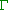. The body left to its own resources tends to pass to the state with more statistic weight. But it is done to use not the numberbut its logarithm multiplied by the Boltzmann constant k. The value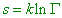(1)
 found in such way is called the entropy of the body” [4, p. 213].   But it appears, these definitions not so clearly describe the entropy and its basic properties. In particular, there exists such one: “Entropy S is the function of state of thermodynamical system characterising the tendency of heat exchange between the system and surrounding medium” [7, p. 311]. It follows from this definition that entropy already defines not the property of conservative system but the heat exchange between the system and environment. As we will see further, this basic nuance more precisely defines the common idea of entropy, although when formalised, this aspect leaves the conception of entropy without its initial phenomenological grounds. Paschenko’s definition  is more relevant to the processes described by today authors concerning the entropy. We can easily show it on some typical examples that most of the authors use to give. In particular, consider the modelling experiment described by R.W. Pohl. “We can carry out an irreversible process very visually. We will spatially separate the process of gas expansion from the processes of heat supply and internal friction. Let us use mentally the device shown in Fig. 1.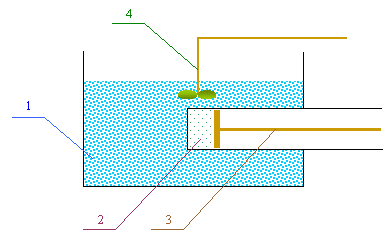Fig. 1. Demonstration of irreversible process by R.W. Pohl [8, p. 435, Fig. 553]: 1 is the water bath, 2 is the cylinder with the gas, 3 is the plunger, 4 is the mixer
 The cylinder filled with the gas is in thermal contact with a large reservoir – e.g., with the water bath whose temperature is T(1) . Together they … form a closed-loop system – the system protected from the heat exchange with the environment. This amount of gas has to expand quasistatically and isothermally. It takes the energy from the water bath as the heat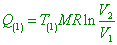(2)
 (where M is the mass of gas, R is the gas constant, V1 , V2 are the initial and final volumes of gas – Authors). With the help of plunger, this energy fully turns into the work, i.e. A = Q(1) . This work makes the mixer moving. So it is spent for forming vortexes and friction, i.e. turns into heat and returns to the water bath. In the end of this irreversible process, the state of water bath remained, thus, absolutely unchanged. There changed only the state of this portion of gas” [8, p. 435].   First of all, let us draw our attention that in the initial state, the temperatures of water bath and gas were inequal, and this is the point from which we would have to start speaking of gas expansion when contacting with the bath. In its turn it means that there was supplied to the bath some quantity of heat that during the experiment was redistributed between the bath and expanding mass of gas. Consequently, first, the system already is non-conservative and it is incorrect to separate the heat supply from the gas expansion. Second, the gas expansion was caused by heating. Without this heating, the expansion would not take place. A definite thermal energy was spent for this heating, and only its part returned into the gas-expansion work. As the result, the temperature of gas will differ from that initial, so the gas will have other energy. And this temperature in no way will return through the mixer to the bath, even with 100 % efficiency. Third and the main, the plunger expanded not only because it was affected by gas in cylinder but also because the gas on another side of plunger had other temperature and pressure. Just the difference of pressures moved the plunger. From this, the work of plunger depended on the parameters of medium out of conservative system. From this it follows, again, that Pohl’s system is non-conservative and in this system were initially created the conditions that disbalanced it, then the system returned to its equilibrium. Thus, we may not consider this scheme as an example of conservative system in which irreversible processes occur. If the initial equilibrium in the system could be disturbed and then restored in some way, this is already a non-irreversible process. Possibly, Pohl’s example is not of most felicitous, though other authors give it in different versions. Consider another, also conventional, example given by L.I. Sedov. “Suppose, there are two infinitesimal volumes I and II of incompressible liquid with same pressures and different temperatures. Let the temperature of the volume I be T1 and temperature of the volume II be T2 (T2 > T1). If we put these volumes in touch, they will exchange heat, and at each moment of time to each volume I and II we can attribute a definite value of temperature (as the volumes I and II are small). The heat transfer from II to I is irreversible, as in accordance with the second law of energetics the heat can be transferred without loss of energy only from the particle with temperature T2 to the particle with temperature T1 , if T2 > T1 . Denote as dQ the positive quantity of heat that passes from the particle II to the particle I during the time dt and consider a simple case when the system consisting of an ensemble of particles I and II has no heat exchange with the environment. If the irreversibility relates only to the particle-to-particle heat transfer, while the states and processes in each separate particle we can think reversible, for separate particles we can write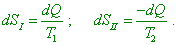(3)
 We can calculate the change of entropy of the whole system I + II, premising the complete entropy S to be an additive function, i.e.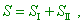consequently,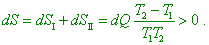(4)
 Thus, though in the considered example there is no external inflow to the system I + II, the entropy of this system grows on the account of irreversible interior process” [9, p. 234]. We can see from this citation that despite passing from the macrosystem to infinitely small volumes and even to separate molecules, the questions to this model remain. First of all, when separating the thermodynamical system into subsystems, these last have to satisfy the concept ‘macroscopic’ [6, p. 535]. Otherwise the statistical type of process will be violated, so the idea of entropy is lost, and the idea of temperature in thermodynamical sense also much changes. If L.I. Sedov considered the energy transfer between two specific particles of the volumes I and II, he has to consider the kinetic temperature of specific particles and account that the total energy of particles remains during their interaction. From this, when constant temperature, no energy transfer can take place, and no addition of entropies. This is possible only in statistic processes and, as we will show below, in far from every thermodynamic process. Thus, all Sedov’s computations lose their physical meaning. Second, Sedov considers the case when the temperature of systems I and II was different and confines his consideration exceptionally to heat transfer. With it, formation of local heterogeneity appears excluded from consideration. Yes, indeed, heat is passed strongly from the heated body to that colder, the second law of energetics is absolutely true in this. But should the processes of interaction be limited to heat transfer, in the initially conservative system we would be unable to create the disbalance whose consequences Sedov analyses. Third, if in one of volumes the temperature was changed in such or other way, this automatically means that the heat was introduced or taken away with help of some external sources whose presence Sedov negates, while it is incorrect to negate them. This distorts the phenomenology of exchange interactions between the thermodynamical subsystems. Fourth, the process described by Sedov is not thermostatic. If speaking of specific particles, in the interaction the kinetic temperature of a more energetic particle will fall, and that of more energetic particle will grow. The energies will average but the total energy of the system of particles will conserve. But if we consider the heat transfer from the view of macrosystems, the initial temperatures of volumes will not remain. Consequently, we may not consider this process as isothermal, so it would be incorrect to write the equalities as (3) and (4). And when considering the heat transfer properly, we would need to introduce the thermal capacity of liquid, to account the temperature change of each volume, the possibility of volumes expansion etc. In particular, in case of ideal gas, we would have to proceed from the expression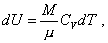(5)
 where CV is the molar heat capacity in the constant volume and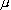is the molecular mass of gas. “In case of ideal gas, (5) is true for any process” [10, p. 201]. For liquids it would be more complicated, as we would need to account the Van der Vaals forces. Then the modelling equations will be other, and the result will be in no way related to the concept of entropy. So we can conclude, in this case there was studied the conservative system exceptionally in the meaning that provides the study of heat transfer from the heated body to that colder, not the whole amount of phenomena providing the heat turnover in the nature. To study the whole amount of phenomena, Sedov’s system is not conservative and may not be applied to calculate the entropy. At the same time, this example is interesting, as it shows the necessity to analyse thoroughly the concept of statistic weight of the state. And on the other hand, this example points the difficulties with the classification of irreversibility of thermodynamic processes from the view, how can we extend the concept of entropy onto the global-scale physical phenomena. To make a little clearer the concept of statistic weight of the system, consider the typical example, how Landau calculated the statistic weight. “We can get an idea, how improbable is the body’s spontaneous deviation from equilibrium, at least on the example when gas expands into a void. Let the gas be initially located in one half of the vessel (here again we see the statement of problem of a prematurely disbalanced system – Authors) divided by a partition into two equal parts. When the gap in partition opened, the gas will spread equally in both parts of vessel. But the reverse transition of gas to one half of vessel will never take place without an extraneous intervention” [4, p. 211]. Here we will interrupt the citation in order to point: when defining the nature of irreversibility of processes, Landau, just as many other authors, intentionally excludes from the consideration the physical processes that lead to the heterogeneity, although these are the same spontaneous processes. Further we will especially consider some such processes. But here, to give an example, let us look at the scheme with three vessels shown in Fig. 2.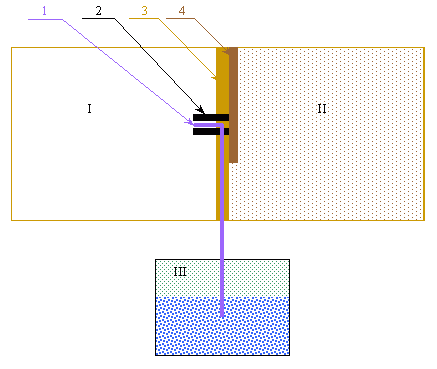Fig. 2. The scheme of gas throttling from one vessel to another in presence of the third vessel half-filled of liquid: 1 is the capillary from the vessel with a liquid, 2 is the throttle from the vessel II with a gas being under pressure p2  to the vessel I with the gas under pressure p1 (p2 > p1), 3 is the partition, and 4 is the damper
 We can see from this scheme that when the damper opened, the gas from second vessel, going through the throttle, will entrain the liquid from the third vessel to that first. With it we will see the pressures not equalising in all three vessels but falling in the third vessel, and the first vessel will be partially filled by liquid. At the same time, the gas expansion in the third vessel will cause the fall of its temperature. This only from one side will cause the balance of system, but from another, it will not equalise the temperatures and pressures, to which all authors bring their schemes excluding from consideration all processes that caused the disbalance. We should notice, this feature is very important in the view that the concept ‘entropy’ is extended onto the evolution of processes in the universe. Following this habitual separation of processes, entropy cannot reflect completely the state of physical object, as it does not reflect a full amount of physical properties of the object, only the properties determining the system’s tending to the thermodynamical equilibrium. While the equilibrium can be achieved due to balance of action and counteraction – and we see this first excluded from the definition of entropy. Let us continue citing Landau. “It is easy to clear the cause of it (impossibility of reverse transition – Authors) by a simple calculation. Each molecule in its motion in average spends same time in both parts of vessel; we can say, the probability of its location in each half of vessel is ?. If we can think the gas ideal, its molecules move independently from others. So the probability to find two given molecules at the same time in one half of vessel is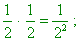(6)
 and the probability to find all N molecules of gas in one part is 2 -N  … Alike enormously small number (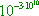) expresses, as calculation shows, the probability of spontaneous transfer of only 1 erg of heat from a body with temperature 0 oC to a body whose temperature is by 1 o higher” [4, p. 211- 212]. Here we encounter a mere mathematisation of physical processes, when for the sake of some simplification introduced into modelling, the phenomenology is fully emasculated and mathematics is reduced to an absurdity. First of all, a simplified scheme of rectangular box has been taken without any grounds. A least change of this scheme will change the probability. Next, the model of ensemble of particles has been reduced to two particles that do not interact with each other. If this was admissible for two particles, this is clearly inadmissible for ideal gas having many particles. The ideality is not when the particles do not interact but when the between-collisions time is much longer than the time of collision, and the main, when the collisions are elastic. This is the ideality. And when we account the interaction, the modelling scheme and methods to study it will fully change. Just so in gas medium not the laws of mathematical probability work but the law of gradient of pressure, as it more accurately describes the direction of flows. The same with a small probability of heat transfer from a low-energy molecule to a high-energy molecule. Here the laws of simple probability of event are also inapplicable. If speaking specifically of two particles, the energy transfer will be determined here by the laws of mechanics, where the energy is not ‘lost’ either ‘got’ but is redistributed between two interacting particles. And this is reflected at the macro-scale level as the energy redistribution in the aggregate thermodynamical system. Another thing that according to the statistical distribution of molecules in energy, the interaction of high-energetic molecule from a colder part of volume with a low-energetic molecule from a heated part of volume is possible. But this is not a heat transfer from a colder body to that heated, as we have to consider this process not separately from other interactions but as a set of processes taking place in the ensemble of molecules. And in this ensemble the situation is absent when all molecules have definite speeds during some interval of time. Well, the statistical curve only accurate to fluctuations registers the number of molecules having definite speeds but does not attribute these speeds to those molecules. While in order, a definite number of molecules with definite parameters to have interacted, they have to hit simultaneously to the common points, keeping their energies during some interval of time before and after the interaction. But namely this is impossible, just as impossible is to make interacting only the molecules of our interest and non-interacting – those that are out of our interest. Those out of our interest will interact more frequently, as in the heated volume there is more high-energetic molecules. This calculation already will not reflect the probability that Landau showed, and any probability of energy transfer from an ensemble with lower-energetic molecules to that with higher-energetic molecules will be absent. But the main mistake of calculations of such kind is, again, that they disregard the pre-history, where the disbalance originated. Should the process have one direction, we would not be able to conduct the experiments on diffusion, as, accurate to small fluctuations, such processes would be impossible because of their irreversibility. Just so all authors specially stipulate, it is impossible to create such disbalanced situations without an external source of energy. But where from such external source can appear, if the energy concentration in some limited volume is absent? Chemical reactions? Well, what are they? Two or several molecules joined their exterior electrons and it caused the energy emission. Due to this, the result of reaction has been heated. Consequently, the energy that caused the heating of two initial molecules was located within the same molecules, not out of them. So it appears that the resulting temperature of some ensemble of molecules can grow, if we will not confine ourselves exceptionally to linear processes but in forming gnosiologic conclusions will account the whole amount of phenomena, in that number chemical, nuclear, thermonuclear etc. But then we will need looking at the concept ‘entropy’ not from the view of artificially selected and ‘combed’ experiments but from view of the whole complex of phenomena that reflect the energy balance both in a local volume and in the universe on the whole.

Contents: / 18 / 19 / 20 / 21 / 22 / 23 / 24 / 25 / 26 / 27 /